# Statistics - math word problems

1. Two diggers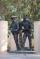There are two diggers. One digger digs a pit 46 hours second gidding 2 times faster. a) how long took to dig a pit second digger b) how long took dig together
2. Target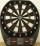Peter, Martin and Jirka were fire in a special target, which had only three fields with values of 12, 18 and 30 points. All boys were firing with the same number of arrows and all the arrows hit the target, and the results of every two boys differed in on
3. Pedestrian up-down hill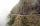Pedestrian goes for a walk first at plane at 4 km/h, then uphill 3 km/h. Then it is in the middle of the route, turns back and goes downhill at speed 6 km/h. Total walk was 6 hours. How many kilometers went pedestrian?
4. Garden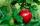Father dig up the garden in 9 hours. Son in 13 hours. How many hours take dig up the garden together?
5. Exam average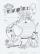Average of marks that have on the certificates students of 8.A class in mathematics is exactly 2.45. If we did not add 1 and 3 of sutdents Michael and Alena, who arrived a month ago, it would average exactly 2.5. Determine how many students have class 8
6. Alcohol 2Two types of alcohol one 63% and second 75% give 20 liters of 69% alcohol. How many liters of each type are in the mixture?
7. MPG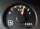252 miles a week fills up twice a week. Each fill is 38 gallons. What is gas mileage of the car?
8. Bases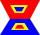The length of the bases trapezium are in ratio 4:5. Length of midline is 15. How long are the bases of a trapezoid?
9. Painter Pavel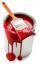Painter Pavel painted the fence for 16 hours and painter Petr the same fence painted for 13 h. How long should take painted the fence together?
10. Fire tank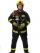Whole fire tank was discharged once in 5 days by first out by second in \$n days. Once firefighters pumped out 6/13 of water out from the tank and then let the water flow out both drain. How long take empty the tank?
11. Blueberries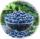Marie earns 3 liters of blueberries for 3.4 hours, Peter 2.4 liters for 2.9 hours. How long have earns together one liter?
12. Two valves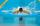Water fill the pool by two valves for 11 days. After 7 days the first valve was stopped and the second valve fill pool for 7 days. How many days took to fill the pool each of the valves individually?
13. Machines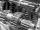In the workshop are three machines. The first is treated 250 parts for 4.7 hours, the second is treated 300 parts for 1.9 hours and the third is treated 230 parts for 4.6 hours. How long will take to make 3100 parts if worked all three machines at the
14. Three workers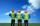Three workers A, B, C have to work on a specific task. Workers A and B completed whole task in 14 days, B together with C for 23 days, A together with C for 13 days. How long it would take to complete the task every one of them alone? How long it would
15. See harmonics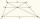It is true that the size of the central segment of any trapezoid is the harmonic mean size of its bases? Prove it. Central segment crosses the intersection of the diagonals and is parallel to the bases.
16. Average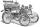What is the average speed of the car, where half of the distance covered passed at speed 66 km/h and the other half at 86 km/h.
17. Center of gravityThe mass points are distributed in space as follows - specify by coordinates and weight. Find the center of gravity of the mass points system: A1 [14; -2; 5] m1 = 10.2 kg A2 [-2; -16; 7] m2 = 13.6 kg A3<
18. Inflow - outflow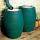The tank will fill by inflow for 143 minutes and empties by outflow for 150 minutes. How long take to fill tank if it is also opened the inflow and outflow?
19. Statistics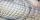The sum of all deviations from the arithmetic mean of the numerical sequence 4, 6, 51, 77, 90, 93, 95, 109, 113, 117 is:
20. Parquet floor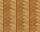Three workers laid parquet floor for 2.301 hour. The first one would do this work alone 7 hours, the second in 6 hours. For how many hours would fulfill this work the third worker, if he worked alone?

Do you have an interesting mathematical word problem that you can't solve it? Submit math problem, and we can try to solve it.

We will send a solution to your e-mail address. Solved examples are also published here. Please enter the e-mail correctly and check whether you don't have a full mailbox.

Please do not submit problems from current active competitions such as Mathematical Olympiad, correspondence seminars etc...